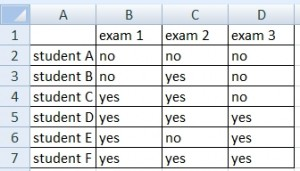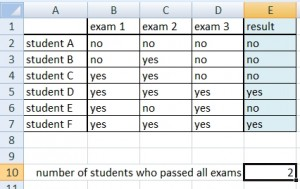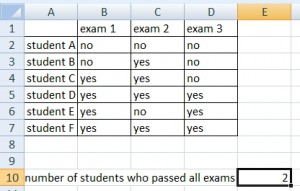# Number of rows that (not) contain a specific value

I prepared two ways to solve this problem. The first uses values in a new column and the second uses array formula. Both have their advantages.

## Example

Table in the picture below contains information about the success of students making 3 exams. Value “Yes” means he passed, value “No” means he did not pass. Student should have value “Yes” in each column to pass all exams.How many students pass all of exams? The question is, how to count rows having value “Yes” in column B or C or D.

### Solution 1

If we could add values into the table, we will create a new column in which will Excel decide about values. Formula in new column is following:

`=IF(OR(B2="no",C2="no",D2 ="no"),"no","yes")`

We can use this formula as an alternative (the result is the same):

`=IF(AND(B2="yes",C2="yes",D2 ="yes"),"yes","no")`

Result:Now it remains only to count value “yes” in column E. Formula for that:

`=COUNTIF(E2:E7,"yes")`

Finish:### Solution 2

This is the way without auxiliary column. The calculation consists of one array formula.

`{=COUNTA(A2:A7)-SUM(IF(MMULT(IF(B2:D7 ="no",1,0),{1;1;1})>0,1,0))}`

Result:1.2.